Courses

# Kirchhoff's Rules Class 12 Notes | EduRev

## Class 12 : Kirchhoff's Rules Class 12 Notes | EduRev

The document Kirchhoff's Rules Class 12 Notes | EduRev is a part of the Class 12 Course Physics For JEE.
All you need of Class 12 at this link: Class 12

Kirchhoff's Laws for Circuit Analysis

• Before moving on to the statement of Kirchhoff's law, we state some conventions to be followed in circuit analysis :
(i) Direction of conventional current is from high potential to low potential terminal.
(ii) Current flows from high potential node A to low potential node B. if we traverse from point A to B, there is drop of potential; similarly from B to A, there is gain of potential.
• If we traverse from point A to B, there is drop of potential; similarly from B to A, there is gain of potential. If a source of emf is traversed from negative to positive terminal, the change in potential is +E.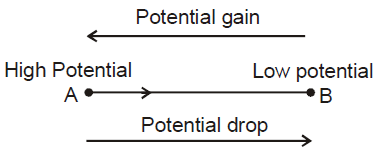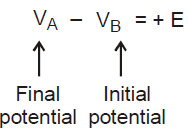• While discharging, current is drawn from the battery, the current comes out from positive terminal and enters negative terminal, while charging of battery current is forced from positive terminal of the battery to negative terminal. Irrespective of direction of current through a battery the sign convention mentioned above holds.
• The positive plate of a capacitor is at high potential and negative plate at low potential. If we traverse a capacitor from positive plate to negative plate, the change in potential is -Q/C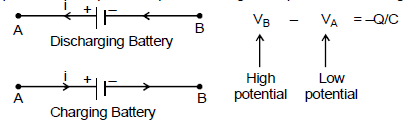• If we traverse a resistor in the direction of current, the change in potential is -IR.
• If we traverse a resistor in the direction opposite to the direction of current, the change in potential is +IR.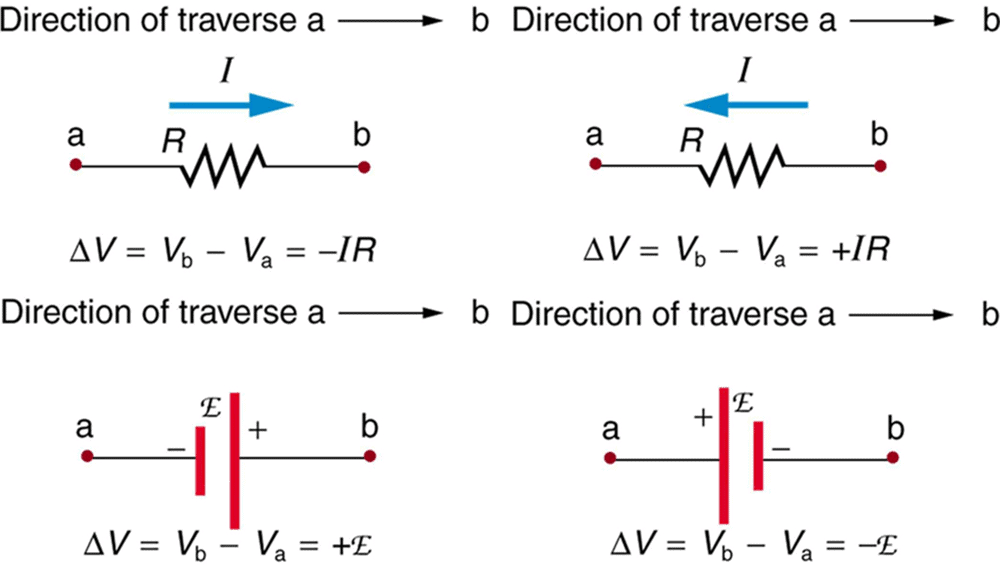• Positive terminal of source of emf is at high potential and negative terminal at low potential. If we traverse a source of emf from the positive terminal to negative terminal, the change in potential is -E.
• If a capacitor is traversed from negative plate to positive plate, the change in potential is Q/C.

The Kirchhoff's Current Law

• Current flow in circuits is produced when charge carriers travel though conductors. Current is defined as the rate at which this charge is carried through the circuit. A fundamental concept in physics is that charge will always be conserved.
• In the context of circuits this means that, since current is the rate of flow of charge, the current flowing into a point must be the same as current flowing out of that point.• Kirchhoff's current law states that for the diagram above, the currents in the three wires must be related by:
I1 + I2 = I3
• It is important to note what is meant by the signs of the current in the diagram - a positive current means that the currents are flowing in the directions indicated on the diagram.

The Kirchhoff's Voltage Law

• The Kirchhoff's voltage law (KVL) states that the algebraic sum of the potential difference around any closed loop of an electric circuit is zero. The KVL is a statement of conservation of energy
• The KVL reflects that electric force is conservative, the work done by a conservative force on a charge taken around a closed path is zero.
• We can move clockwise or anticlockwise, it will make no difference because the overall sum of the potential difference is zero.
• We can start from any point on the loop, we just have to finish at the same point.
• An ideal battery is modelled by an independent voltage source of emf E and an internal resistance r as shown in figure A real battery always absorbs power when there is a current through it, thereby offering resistance to flow of current.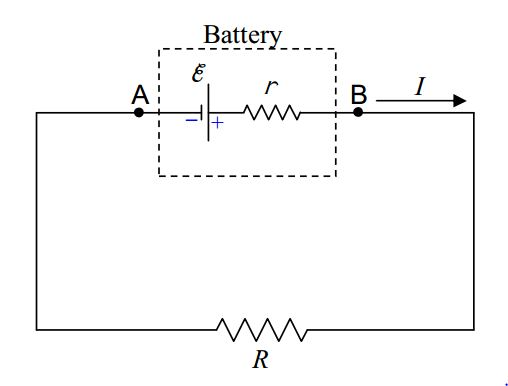• Applying KVL around the single loop in anticlockwise direction, starting from point A, we have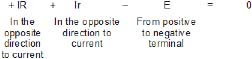Hence,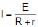Example 1. Find current in the circuit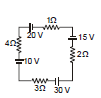Sol. Therefore, all the elements are connected in series

Therefore, current in all of them will be same

let current = I

Applying kirchhoff' s voltage law in ABCDA loop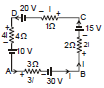10 + 4i - 20 + i + 15 + 2i - 30 + 3i = 0

10 i = 25 ⇒ i = 2.5 A

Example 1. Find the current in each wire applying only kirchhoff voltage law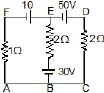Sol. Applying kirchhoff voltage law in loop ABEFA

i1 + 30 + 2 (i1 + i2) - 10 = 0

3i1 + 2i2 + 20 = 0 ...(i)

Applying kirchhoff voltage law in BCDEB

+30 + 2(i1 + i2) + 50 + 2i2 = 0

4i2 +  2i1 + 80 = 0

2i2 + i1 + 40 = 0 ...(ii)

Solving (i) and (ii)

3[-40 -2i2] + 2i2 + 20 = 0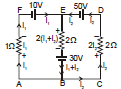-120 - 4i2 + 20 = 0

i2 = - 25 A

and i1 = 10 A

Therefore, i1  i2 = - 15 A

current in wire AF = 10 A from A to E

current in wire EB = 15 A from B to E

current in wire DE = 25 A from D to C

Offer running on EduRev: Apply code STAYHOME200 to get INR 200 off on our premium plan EduRev Infinity!

## Physics For JEE

188 videos|346 docs|307 tests

,

,

,

,

,

,

,

,

,

,

,

,

,

,

,

,

,

,

,

,

,

;